# RD Sharma Solutions For Class 12 Maths Exercise 10.2 Chapter 10 Differentiability

RD Sharma Solutions for Class 12 Maths Exercise 10.2 Chapter 10 Differentiability is provided here. In order to excel in the board exam for Class 12, students can use the solutions of Exercise 10.2 available on the website. This exercise mainly explains the concept of differentiability in a given set.

By practising regularly with the RD Sharma Solutions, students can obtain worthy results in the Class 12 exams. The PDF of solutions to exercise 10.2 of RD Sharma Solutions for Class 12 Maths primarily helps students from the exam perspective.

## RD Sharma Solutions For Class 12 Chapter 10 – Differentiability Exercise 10.2:-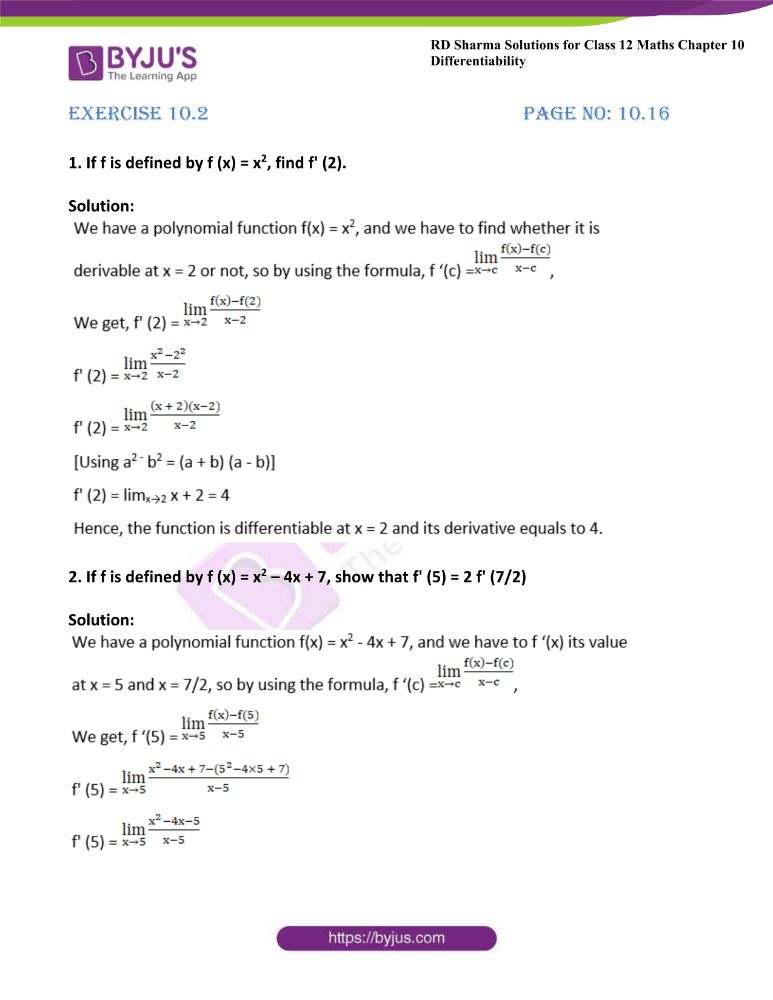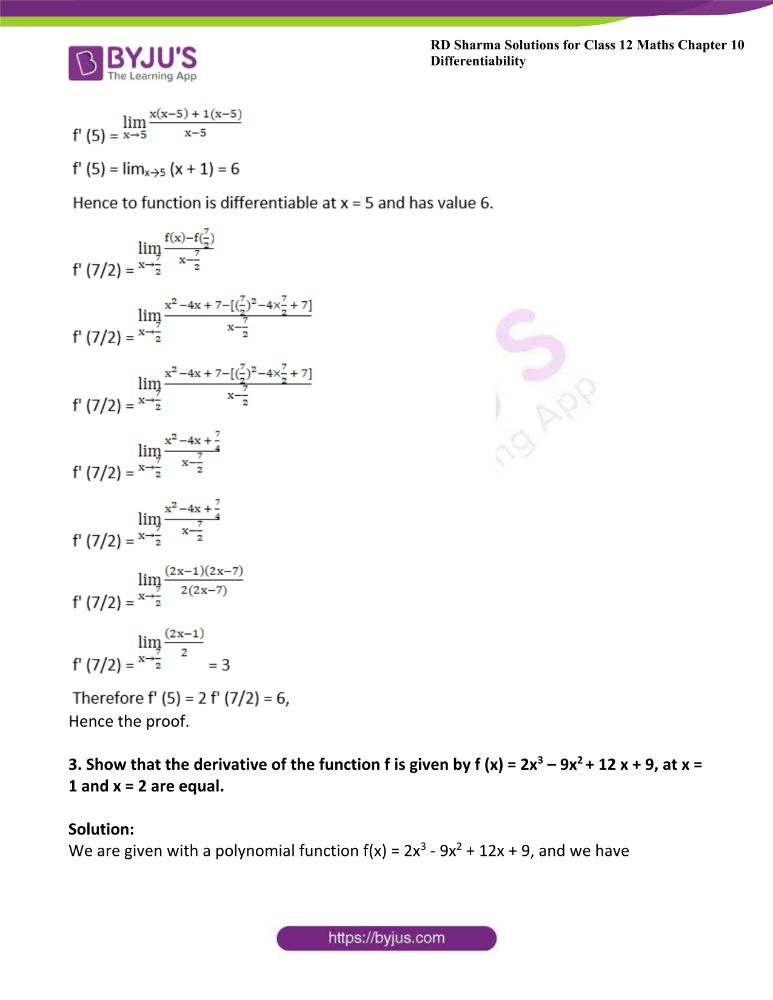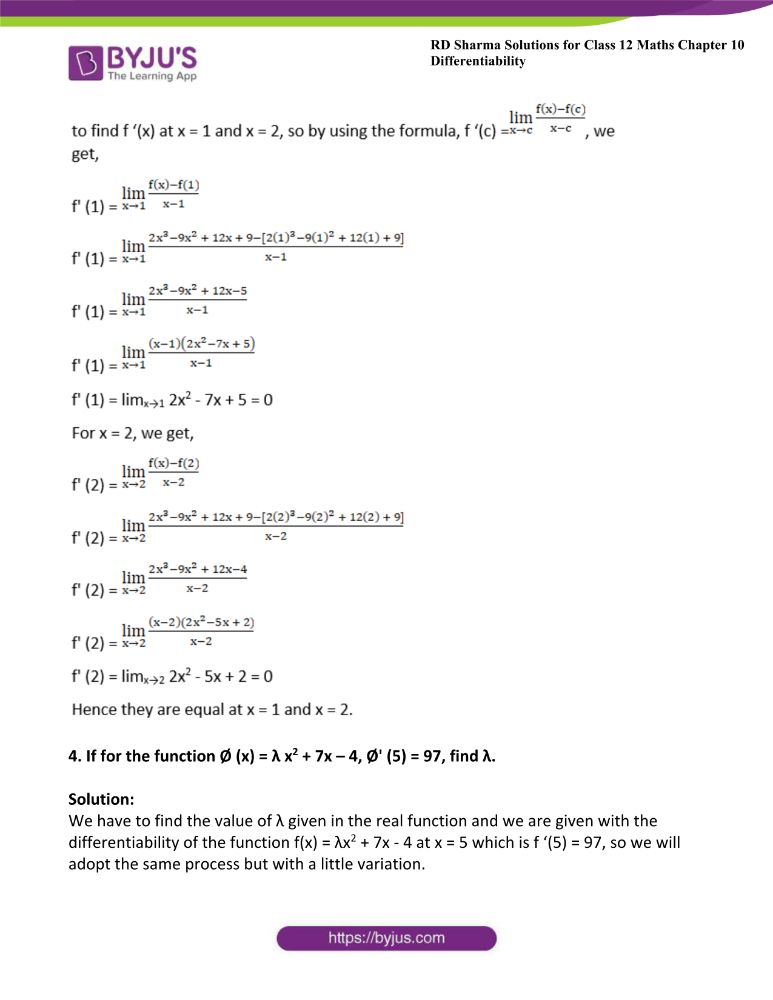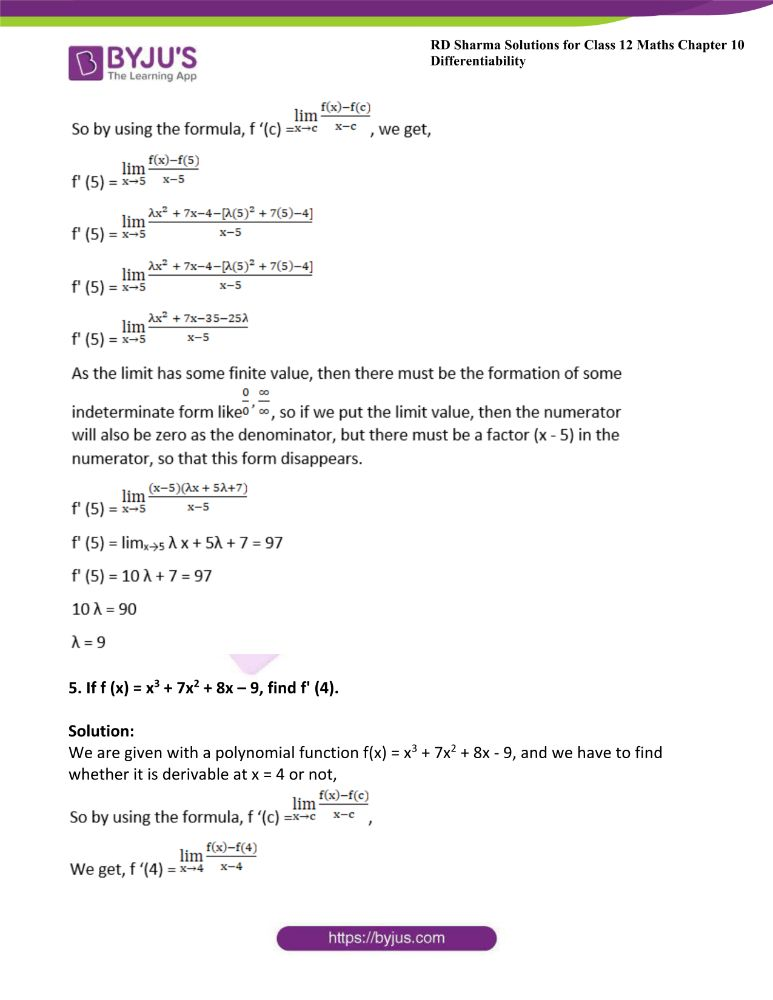### Access another exercise of RD Sharma Solutions For Class 12 Chapter 10 – Differentiability

Exercise 10.1 Solutions

### Access answers to Maths RD Sharma Solutions For Class 12 Chapter 10 – Differentiability Exercise 10.2

Exercise 10.2 Page No: 10.16

1. If f is defined by f (x) = x2, find f’ (2).

Solution: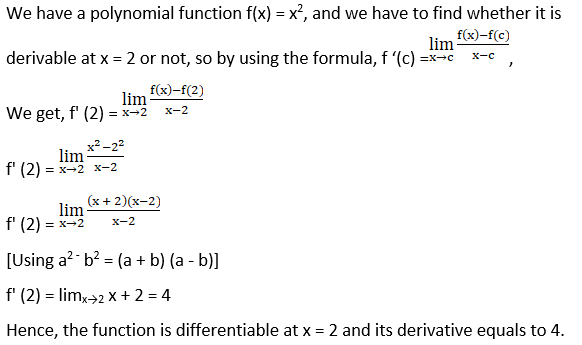2. If f is defined by f (x) = x2 – 4x + 7, show that f’ (5) = 2 f’ (7/2)

Solution: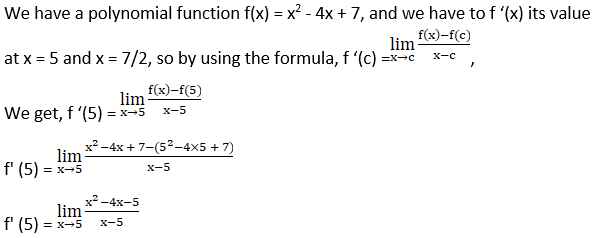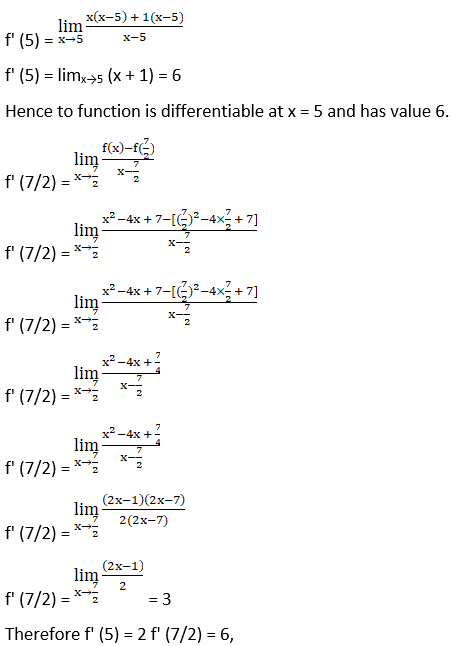Hence the proof.

3. Show that the derivative of the function f is given by f (x) = 2x3 – 9x2 + 12 x + 9, at x = 1 and x = 2 are equal.

Solution:

We are given with a polynomial function f(x) = 2x3 – 9x2 + 12x + 9, and we have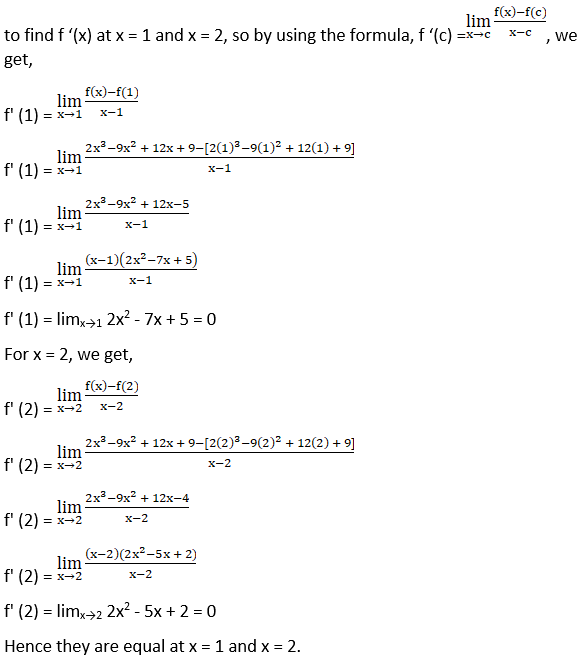4. If for the function Ø (x) = λ x2 + 7x – 4, Ø’ (5) = 97, find λ.

Solution:

We have to find the value of λ given in the real function and we are given with the differentiability of the function f(x) = λx2 + 7x – 4 at x = 5 which is f ‘(5) = 97, so we will adopt the same process but with a little variation.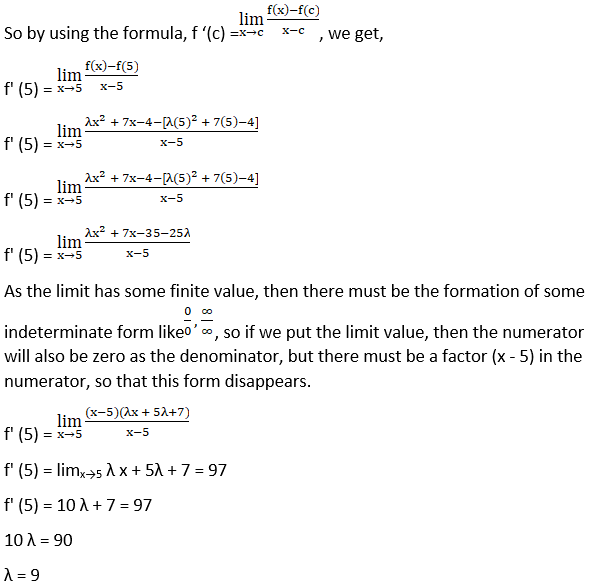5. If f (x) = x3 + 7x2 + 8x – 9, find f’ (4).

Solution:

We are given with a polynomial function f(x) = x3 + 7x2 + 8x – 9, and we have to find whether it is derivable at x = 4 or not,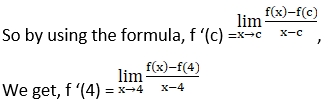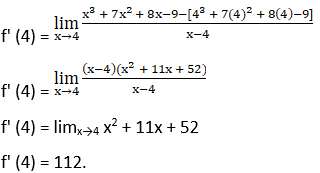6. Find the derivative of the function f defined by f (x) = mx + c at x = 0.

Solution:

We are given with a polynomial function f(x) = mx + c, and we have to find whether it is derivable at x = 0 or not,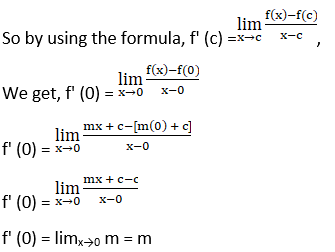This is the derivative of a function at x = 0, and also this is the derivative of this function at every value of x.